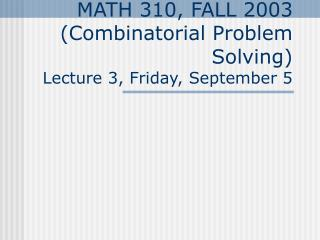DownloadDownload PresentationMATH 310, FALL 2003 (Combinatorial Problem Solving) Lecture 3, Friday, September 5

# MATH 310, FALL 2003 (Combinatorial Problem Solving) Lecture 3, Friday, September 5

Télécharger la présentation## MATH 310, FALL 2003 (Combinatorial Problem Solving) Lecture 3, Friday, September 5

- - - - - - - - - - - - - - - - - - - - - - - - - - - E N D - - - - - - - - - - - - - - - - - - - - - - - - - - -
##### Presentation Transcript

1. MATH 310, FALL 2003(Combinatorial Problem Solving)Lecture 3, Friday, September 5

2. Complete graph Kn. • A graph on n vertices in which each vertex is adjacent to all other vertices is called a complete graph on n vertices, denoted by Kn. K20

3. Some complete graphs • Here are some complete graphs. • For each one determine the number of vertices, edges, and the degree of each vertex. • Every graph on n vertices is a subgraph of Kn.

4. Example 2: Isomorphism in Symmetric Graphs • The two graphs on the left are isomorphic. • Top graph vertices clockwise: a,b,c,d,e,f,g • Bottom graph vertices clockwise: 1,2,3,4,5,6,7 • Possible isomorphism:a-1,b-5,c-2,d-6,e-3,f-7,g-4.

5. Example 3: Isomorphism ofDirected Graphs • Some hints how to prove non-isomorphism: • If two graphs are not isomorphic as undirected graphs, they cannot be isomorphic as directed graphs. • (p,q) –label on a vertex: indegree p, outdegree q. • Look at the directed edges and their (p,q,r,s) labels! (2,3) 1 (p,q) (r,s) (p,q,r,s) 2 3 e

6. 1.3. Edge Counting • Homework (MATH 310#1F): • Read 1.4. Write down a list of all newly introduced terms (printed in boldface) • Do Exercises1.3: 4,6,8,12,13 • Volunteers: • ____________ • ____________ • Problem: 13. • News: • Please always bring your updated list of terms to class meeting. • Homework in now labeled for easier identification: • (MATH 310, #, Day-MWF)

7. Theorem 1 • In any graph, the sum of the degrees of all vertices is equal to twice the number of edges.

8. Corollary • In any graph, the number of vertices of odd degree is even.

9. Example 2: Edges in a Complete Graph • The degree of each vertex of Kn is n-1. There are n vertices. The total sum is n(n-1) = twice the number of edges. • Kn has n(n-1)/2 edges. • On the left K15 has 105 edges.

10. Example 3: Impossible graph • Is it possible to have a group of seven people such that each person knows exactly three other people in the group?

11. Bipartite Graphs A graph G is bipartite if its vertices can be partitioned into two sets VL and VR and every edge joins a vertex in VL with a vertex in VR Graph on the left is biparite.

12. Theorem 2 • A graph G is bipartite if and only if every circuit in G has even length.

13. Example 5: Testing for a Bipartite Graph • Is the graph on the left bipartite?